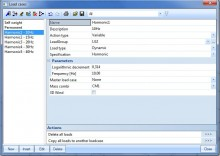# sens.11 - Seismic harmonic load time-history analysis

sens.11

## Highlights

 For the harmonic load, the frequency and the damping is defined. Response of a structure to a harmonic or seismic load and direct time integration can be calculated. The calculation under seismic load is used, amongst others, for simulating earthquakes; the spectra of the EC 8, DIN 4149 (German standard), SIA 261 (Swiss standard) and the Turkish standard are available by default and can be manually extended. The modal participation factors are indicated. For both analyses, the results can be combined with the results from a static calculation.

##Harmonic calculation

• User input of load frequency and overall damping (logarithmic decrement) for each load case
• More than one harmonic load case can be input, each with a different value of frequency
• Results from modal analysis are available: eigen frequencies, mass participation factors and eigenmode shapes
• The same menus as for static load cases are available to view numerical or graphical results: displacements, internal forces and reactions

## Seismic calculation

• Spectra of the EC 8 and national codes: Austria, Czech Republic, France, Germany, India, Italy, Slovakia, Romania, Switzerland, and U.S. are available by default
• The Netherlands standard NPR 9998 is also included.
• Additional spectrum can be defined using table input (copy/paste from Excel is also possible).
• Type of modal superposition: SRSS or CQC method can be selected
• Accidental eccentricity or torsion can be taken into account for buildings
• Automatic generation of Newmark seismic combinations
• Results from modal analysis are available: eigen frequencies, mass participation factors and eigenmode shapes
• The same menus as for static load cases are available to view numerical or graphical results: displacements, accelerations, internal forces and reactions

## Direct Time Integration

• This can be used for different purposes, for example: harmonic loads, explosions, …
• You have to input a dynamic function which presents the frequency in function of the time.

## Vortex shedding: Von Karman vibration analysis

The transverse vibration of cylindrical structures modelled as 1D elements due to wind can be examined

• The effect is only taken into account if the critical wind velocity calculated is between the minimum and maximum value
• It is possible to specify the length of the structure where the Von Karman effect can occur.
• For each geometric node of the structure, it is possible to relate a length of the cylinder to the node.
• The effect can occur over the entire height of the structure however, when there are specific obstacles on the surface of a chimney for example, these obstacles will hamper the formation of the vortices and thus reduce the Von Karman effect.

## Equivalent Lateral Forces

The seismic Equivalent Lateral Forces (ELF) analysis is the most well known method for the seismic analysis of structures. Although it is quite conservative, its simplicity makes it a very popular method for seismic design.

• The ELF method is a static analysis method.
• It requires the input of some data related to dynamic analysis: masses and at least one combination of mass groups.
• The calculation is based on the distribution of masses in the structure.
• The calculation of storey forces is based on the definition of storeys as well as on the reduced system, which must therefore be defined.

### Calculation of the Equivalent Lateral Forces

• The user is able to select from methods for calculation of equivalent lateral forces.
• These method are in compliance with the European and American standards - EN 1998 and ASCE 7-10.
• ELF are calculated in the background after a modal analysis.
• Calculated equivalent lateral forces are applied as one concentrated force at the mass centre of each storey.

Possibility to select how the acceleration is distributed in the building:

• Linear distribution of accelerations (EN 1998-1 clause 4.3.3.2.3 eq. (4.11) )
• Polynomial distribution of accelerations (ASCE 7-10 12.8.3)
• Distribution of accelerations from eigenshape (EN 1998-1 clause 4.3.3.2.3 eq. (4.10) )

### Application of the storey forces to the model

• The calculated storey forces are applied to the structure using the reduced system.
• The transformation matrices of the IRS method make it possible to "smear" the concentrated storey forces in such a way that the resultant of each storey force is applied at the mass centre of the corresponding storey. It is therefore not necessary to define diaphragms to apply storey forces.
• The loads are, however, applied in a distributed way to the entire storey, hence avoiding any numerical singularity, as would be the case if point loads would be applied in a conventional way.

### Results

All standard result output can be used in SCIA Engineer, without any restriction. Also, because it is a static load case, none of the issues related to the loss of sign due to the modal superposition apply here.

### Brief comparison of ELF and response spectrum method

Equivalent lateral forces

Pros

• easy to use, intuitive
• static analysis, which implies the following advantages:
• results easy to check
• signed results &amp; concomitant results

Cons

• limited to regular buildings (acceptance criteria defined in the design codes)
• only fundamental mode is taken into account
• conservative (in applicable cases)

Response Spectrum Method (modal superposition)

Pros

• applicable to all types of buildings (including irregular ones)
• multiple modes taken into account
• less conservative than ELF, more realistic

Cons

• requires understanding of modal analysis
• only elastic linear analysis (2nd order possible)

Required modules:

• sens.10
Included in Edition:
Professional
Expert
Ultimate